Definitions of Square Dance Calls and Concepts

Index -->  Plus  |  A1  |  A2  |  C1  |  C2  |  C3A  |  C3B  |  C4  |  NOL  |
Definitions (Text Only) -->  Plus  |  A1  |  A2  |  C1  |  C2  |  C3A  |  C3B  |  C4  |  NOL  |
 Find call:

Static Set \$B\$+\$i(B (\$B\$^\$?\$O(B Static Set \$B\$+\$i(B Heads Step into the Center \$B\$r\$7\$FF@\$i\$l\$k(B formation) \$B\$^\$?\$O8~\$+\$\$9g\$C\$?%+%C%W%k\$N4V\$GD>3Q\$K\$J\$C\$F\$\$\$k(B Wave \$B\$+\$i!%(B

1. Centers \$B\$^\$?\$O;X<(\$5\$l\$??M\$,(B Arm Turn 3/4 \$B\$r\$7\$F(B,
2. \$BA40w\$G(B Triple Cross \$B\$r\$7(B,
3. Peel Off.

\$BJ?9T\$J(B Two-Faced Line \$B\$G=*\$o\$j\$^\$9!%(B Spin The Pulley \$B\$O(B 3 \$B%Q!<%H\$N%3!<%k\$G\$9(B.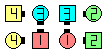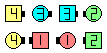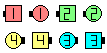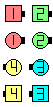Spin The Pulley\$B\$NA0(B Centers Arm Turn 3/4(1/3)\$B\$N8e(B Triple Cross(2/3)\$B\$N8e(B Peel Off\$B\$N8e(B (\$B=*\$o\$j(B)

Spin The Pulley But anything [C3A]: Spin The Pulley \$B\$r\$7\$^\$9\$,(B, Peel Off \$B\$r(B anything call \$B\$G(B Replace \$B\$7\$^\$9!%(B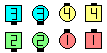Spin The PulleyBut Checkmate\$B\$NA0(B Centers Arm Turn 3/4\$B\$N8e(B Triple Cross\$B\$N8e(B Checkmate\$B\$N8e(B (\$B=*\$o\$j(B)

anything The Pulley [C3A]: anything call \$B\$r\$7\$F(B, Finish a Spin The Pulley (Triple Cross, Peel Off) \$B\$r\$7\$^\$9!%J?9T\$J(B Two-Faced Line \$B\$G=*\$o\$j\$^\$9!%(B

anything The Pulley But anything [C3A]: \$B;O\$a\$N(B anything call \$B\$r\$7\$F(B, Triple Cross \$B\$r\$7(B, 2 \$BHVL\\$N(B anything call \$B\$r\$7\$^\$9!%(BCALLERLAB definition for Spin The PulleyChoreography for Spin The PulleyComments? Questions? Suggestions?